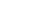# Unbiased in Statistics: Definition and Examples

Statistics Definitions > Unbiased

You may want to read about bias first: What is bias?

Contents:

1. Overview
2. Unbiased estimators
3. MVUEs

## What does it mean to be Unbiased in Statistics?

Watch the video for an overview:

Watch this video on YouTube.

In daily life, we use the word “bias” to mean that there is “…a tendency to believe that some people, ideas, etc., are better than others that usually results in treating some people unfairly” (Merriam Webster). In statistics, the word bias — and its opposite, unbiased — means the same thing, but the definition is a little more precise:

If your statistic is not an underestimate or overestimate of a population parameter, then that statistic is said to be unbiased.

## What can I do to Ensure Unbiasedness in my Data or Sampling Distribution?

There are many steps you can take to try and make sure that your statistics are unbiased and accurately reflect the population parameter you are studying:

• Take your sample according to sound statistical practices. For more information on different sampling types and the advantages and disadvantages of each, see: Sampling Techniques
• Avoid measurement error by making sure data is collected with unbiased practices. For example, make sure any questions posed aren’t ambiguous.
• Avoid unrepresentative samples by making sure you haven’t excluded certain population members (like minorities or people who work two jobs).

One famous example of an unrepresentative sample is the literary digest voter survey, which predicted Alfred Landon would win the 1936 presidential election. The survey was biased, as it failed to include a representative sample of low income voters who were more likely to be democrat and vote for Theodore Roosevelt.

## What is an Unbiased Estimator?

An unbiased estimator is an accurate statistic that’s used to approximate a population parameter. “Accurate” in this sense means that it’s neither an overestimate nor an underestimate. If an overestimate or underestimate does happen, the mean of the difference is called a “bias.”

In more mathematical terms, an estimator is unbiased if:That’s just saying if the estimator (i.e. the sample mean) equals the parameter (i.e. the population mean), then it’s an unbiased estimator.
You might also see this written as something like “An unbiased estimator is when the mean of the statistic’s sampling distribution is equal to the population’s parameter.” This essentially means the same thing: if the statistic equals the parameter, then it’s unbiased.

A more formal definition for the bias (i.e. the difference between the actual and the estimate) is:
For observations X = (X1, X2,…,Xn) based on a distribution having parameter value Θ, and for d(X) an estimator for h(Θ), the bias is the mean of the difference d(X) – h(Θ), i.e.,
bd(Θ) = EΘd(X) – h(Θ).

Any estimator that is not unbiased is called a biased estimator.

## Getting Unbiased Estimators

You can obtain unbiased estimators by avoiding bias during sampling and data collection.

For example, let’s say you’re trying to figure out the average amount people spend on food per week. You can’t survey the whole population of over 300 million, so you take a sample of around 1,000. You find that the average amount people spend per week is \$70 per person. Is this an unbiased estimator? Possibly. It all depends on how you took your sample. For example:

• Were your questions unbiased? For example, an ambiguous question like “How much do you spend on groceries a week?” might seem simple enough. But some people could take this to mean “How much did you spend this week on groceries?” (if it’s the middle of the month, people might spend less) or “How much money did you spend on your household groceries this week?” (be clear that you’re asking per person, not per household.
• Was your sample chosen in an unbiased way (i.e. a simple random sample).
• Have you excluded any population members? For example, if you are performing an internet survey, you may be excluding the poorest 25% of people who do not have internet.

## Minimum Variance Unbiased Estimator(MVUE)

When you take multiple samples from a population, each of those samples will (probably) have different statistics: a slightly different mean or standard deviation/variance. The MVUE is the statistic with the lowest variance.

There isn’t a simple formula to find the MVUE, and it may not actually exist for your samples. There are two main ways you can find/verify a MVUE; both are quite advanced and require some knowledge of mathematical statistics:

1. Use the Cramer-Rao Lower Bound. This sets a lower bound for the variance. If you can find an estimator that meet this condition, you’ve found the MVUE.
2. Find a sufficient statistic and then use the Rao-Blackwell theorem.

Next: read about more ways bias can seep into your sample. What is Bias?.

## References

Dodge, Y. (2008). The Concise Encyclopedia of Statistics. Springer.
Gonick, L. (1993). The Cartoon Guide to Statistics. HarperPerennial.

CITE THIS AS:
Stephanie Glen. "Unbiased in Statistics: Definition and Examples" From StatisticsHowTo.com: Elementary Statistics for the rest of us! https://www.statisticshowto.com/unbiased/
---------------------------------------------------------------------------Need help with a homework or test question? With Chegg Study, you can get step-by-step solutions to your questions from an expert in the field. Your first 30 minutes with a Chegg tutor is free!